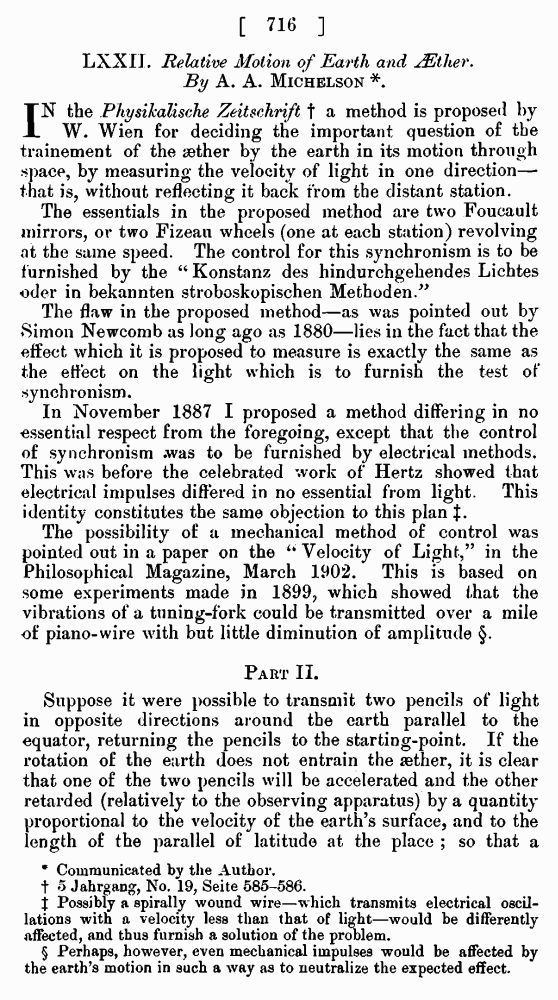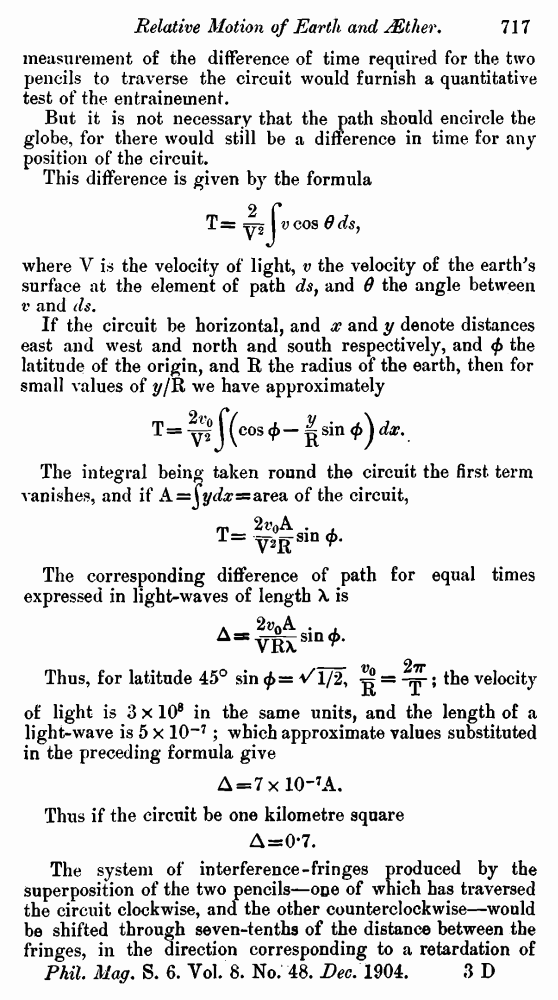﻿ A. A. Michelson. Relative Motion of Earth and AEther // Phil. Mag. S. 6. Vol. 8. No. 48. Dec. 1904.

# A. A. Michelson. Relative Motion of Earth and AEther // Phil. Mag. S. 6. Vol. 8. No. 48. Dec. 1904.

В начало   Другие форматы   <<<     Страница 716   >>>716 717  718  719 LXXII . Relative Motion of Earth and AEther. By A. A. Michelson *. IN the Physikalische Zeitschrift † a method is proposed by W. Wien for deciding the important question of the trainement of the aether by the earth in its motion through space, by measuring the velocity of light in one direction— that is, without reflecting it back from the distant station. The essentials in the proposed method are two Foucault mirrors, or two Fizeau wheels (one at each station) revolving at the same speed. The control for this synchronism is to be furnished by the “Konstanz des hindurchgehendes Lichtes oder in bekannten stroboskopischen Methoden.” The flaw in the proposed method—as was pointed out by Simon Newcomb as long ago as 1880—lies in the fact that the effect which it is proposed to measure is exactly the same as the effect on the light which is to furnish the test of synchronism. In November 1887 I proposed a method differing in no essential respect from the foregoing, except that the control of synchronism was to be furnished by electrical methods. This was before the celebrated work of Hertz showed that electrical impulses differed in no essential from light. This identity constitutes the same objection to this plan ‡. The possibility of a mechanical method of control was pointed out in a paper on the ‘‘Velocity of Light,” in the Philosophical Magazine, March 1902. This is based on some experiments made in 1899, which showed that the vibrations of a tuning-fork could be transmitted over a mile of piano-wire with but little diminution of amplitude §. Part II. Suppose it were possible to transmit two pencils of light in opposite directions around the earth parallel to the equator, returning the pencils to the starting-point. If the rotation of the earth does not entrain the aether, it is clear that one of the two pencils will be accelerated and the other retarded (relatively to the observing apparatus) by a quantity proportional to the velocity of the earth’s surface, and to the length of the parallel of latitude at the place ; so that a * Communicated by the Author. † 5 Jahrgang, No. 19, Seite 585-586. ‡ Possibly a spirally wound wire—which transmits electrical oscillations with a velocity less than that of light—would be differently affected, and thus fnrnish a solution of the problem. § Perhaps, however, even mechanical impulses would be affected by the earth’s motion in such a way as to neutralize the expected effect. measurement of the difference of time required for the two pencils to traverse the circuit would furnish a quantitative test of the entrainement. But it is not necessary that the path should encircle the globe, for there would still be a difference in time for any position of the circuit. This difference is given by the formula where V is the velocity of light, v the velocity of the earth's surface at the element of path ds, and θ the angle between v and ds. If the circuit be horizontal, and x and y denote distances east and west and north and south respectively, and φ the latitude of the origin, and R the radius of the earth, then for small values of y/R we have approximately The integral being taken round the circuit the first term vanishes, and if A=∫ ydx = area of the circuit, The corresponding difference of path for equal times expressed in light-waves of length λ is Thus, for latitude 45°the velocity of light is 3 × 108 in the same units, and the length of a light-wave is 5 × 10–7; which approximate values substituted in the preceding formula give Δ = 7 × 10–7A. Thus if the circuit be one kilometre square Δ=0.7. The system of interference-fringes produced by the Superposition of the two pencils—one of which has traversed the circuit clockwise, and the other counterclockwise—would be shifted through seven-tenths of the distance between the fringes, in the direction corresponding to a retardation of Phil. Mag. S. 6. Vol. 8. No. 48. Dec. 1904. 3 D